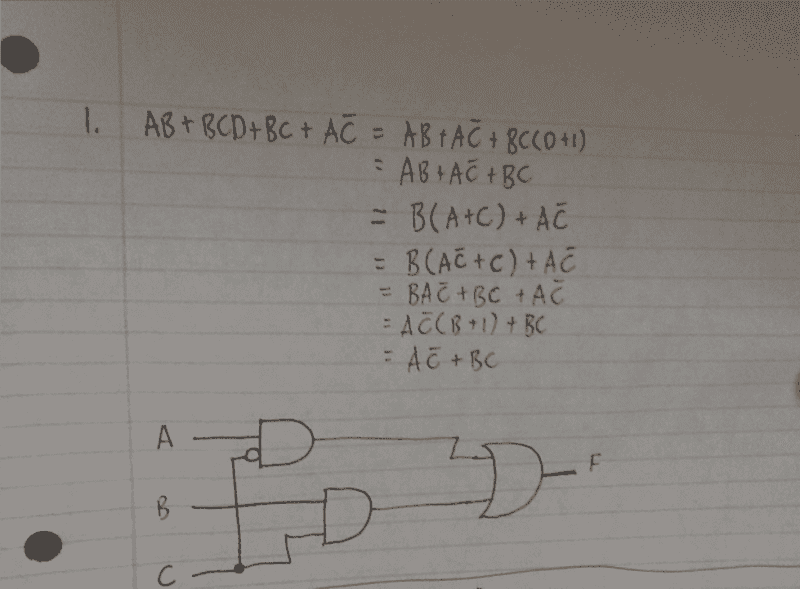# Quick logic questions

Homework Helper

## Homework Statement

1. Reduce the following logical expression to four literals and draw a gate level circuit, which implements the result.

$$AB + BCD + BC + A \bar C$$

2. Simplify the following:

$$(A + \bar B C)(B + C)(B + \bar C)$$

## The Attempt at a Solution

I would just like to verify I understand.

Here is my working for part 1:For part 2 here's what I came up with:

$$(A + \bar B C)(B + C)(B + \bar C)$$
$$= (A + \bar B C)(B + C \bar C)$$
$$= (A + \bar B C)(B + 0)$$
$$= (A + \bar B C)B$$
$$= AB + \bar B B C$$
$$= AB + 0C$$
$$= AB + C$$

I think that's as simple as it can become.

Thank you.

Mentor
A good way to check your work is to draw the Karnaugh Maps and see if the simplest grouping of terms matches your equation manipulations. Can you show us the corresponding K-Maps for this question? :-)

Homework Helper
I have a few more pages before I get to K-Maps today. I actually just started this subject so I don't know much, but I'm working on it.

Mentor
I have a few more pages before I get to K-Maps today. I actually just started this subject so I don't know much, but I'm working on it.

You are going to like K-Maps! :-)

Mentor
BTW, I got a different answer for part 1) using a K-Map. I'd be interested in seeing what you get using that method when you get to it.

Joffan
Note for Q2 that 0C = 0